# Program to check Twisted Prime Program in Java

In this tutorial you will learn about the Program to check Twisted Prime Program in Java and its application with practical example.

In this tutorial, we will learn to create a Java Program to check Twisted Prime Program in Java.

• Twisted Prime Number
• ## Prerequisites

Before starting with this tutorial we assume that you are best aware of the following Java programming topics:

• Java Operators.
• Basic Input and Output function in Java.
• Class and Object in Java.
• Basic Java programming.
• If-else statements in Java.
• Function in java.

# Twisted Prime Number

A given number  called  twisted prime number if it is a prime number and reverse of it also a prime number.

Some Examples of Twisted Prime number 2, 3, 5, 7, 11, 13, 17, 31, 37, 71, 73, 79.

Take a number : “97” is a prime number then
Twisted Prime Number “97” is a prime number
and its reverse “79” is also a prime number.

## Program to check Twisted Prime Program in Java

In this program we would find the given number is twisted prime number or not , first will take the input from the user and check whether it is prime number or not. If it’s prime then we will twist the number and also check it is prime or not.

## Output

Twisted Prime numberNot a prime number.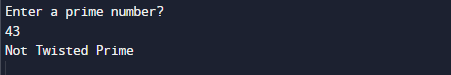In the above program, we have first declared and initialized a set variables required in the program.

• num = it will hold entered number.
• rev = it  will hold reverse or a number
• sum =  for adding values
• count= counting the divisibility
• i= For iteration.

After Declaring  variables we take a prime number from user.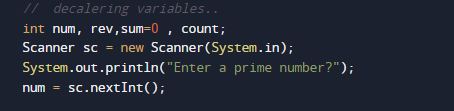First of all we reveres the given number and then check the reverse number is also a prime number or not.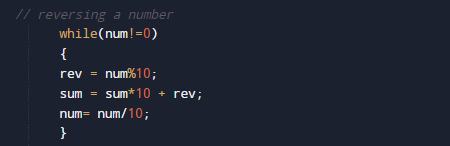In above image we reversing a number then at last we will check the reverse number is also prime or not .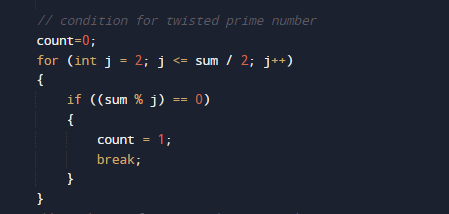if the reverse number is also a prime then we can say the entered prime number is twisted prime number.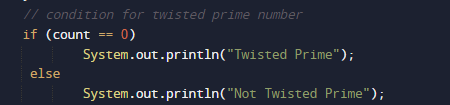else it is not a twisted prime number.

In this tutorial we have learn about the Program to check Twisted Prime Program in Java and its application with practical example. I hope you will like this tutorial.